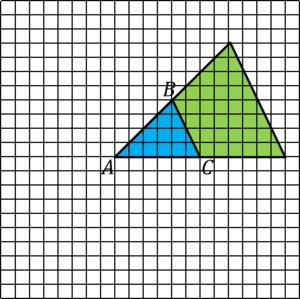# Effects of Dilations on Length, Area, and Angles

Alignments to Content Standards: 8.G.A.3

Consider triangle $ABC$.1. Draw a dilation of $ABC$ with:
1. Center $A$ and scale factor 2.
2. Center $B$ and scale factor 3.
3. Center $C$ and scale factor $\frac12$.
2. For each dilation, answer the following questions:

1. By what factor do the base and height of the triangle change? Explain.
2. By what factor does the area of the triangle change? Explain.
3. How do the angles of the scaled triangle compare to the original? Explain.

## IM Commentary

The purpose of this task is for students to study the impact of dilations on different measurements: segment lengths, area, and angle measure. When a triangle is dilated by scale factor $s \gt 0$, the base and height change by the scale factor $s$ while the area changes by a factor of $s^2$: as seen in the examples presented here, this is true regardless of the center of dilation. While they scale distances between points, dilations do not change angles. While $x$ and $y$ coordinates have not been given to the vertices of the triangle, the coordinate grid serves the same purpose for the given centers of dilation.

Transformations affect all points in the plane, not just the particular figures we choose to analyze when working with transformations. All lengths of line segments in the plane are scaled by the same factor when we apply a dilation. Students can use a variety of tools with this task including colored pencils, highlighters, graph paper, rulers, protractors, and/or transparencies. Task 1681 would be a good follow up to this task, especially if students have access to dynamic geometry software, where they can see that this is true for arbitrary triangles.

## Solution

1. The three dilations are shown below along with explanations for the pictures:

1.The dilation with center $A$ and scale factor 2 doubles the length of segments $\overline{AB}$ and $\overline{AC}$. We can see this explicitly for $\overline{AC}$. For $\overline{AB}$, this segment goes over 6 units and up 4 so its image goes over 12 units and up 8 units.

2.The dilation with center $B$ and scale factor 3 increases the length of $\overline{AB}$ and $\overline{AC}$ by a factor of 3. The point $B$ does not move when we apply the dilation but $A$ and $C$ are mapped to points 3 times as far from $B$ on the same line.

3.

4.The scale factor of $\frac{1}{2}$ makes a smaller triangle. The center of this dilation (also called a contraction in this case) is $C$ and the vertices $A$ and $B$ are mapped to points half the distance from $A$ on the same line segments.

1. When the scale factor of 2 is applied with center $A$ the length of the base doubles from 6 units to 12 units. This is also true for the height which was 4 units for $\triangle ABC$ but is 8 units for the scaled triangle. Similarly, when the scale factor of 3 is applied with center $B$ , the length of the base and the height increase by a scale factor of 3 and for the scale factor of $\frac{1}{2}$ with center $C$, the base and height of $\triangle ABC$ are likewise scaled by $\frac{1}{2}$.
2. The area of a triangle is the base times the height. When a scale factor of 2 with center $A$ is applied to $\triangle ABC$, the base and height each double so the area increases by a factor of 4: the area of $\triangle ABC$ is 12 square units while the area of the scaled version is 48 square units. Similarly, if a scale factor of 3 with center $B$ is applied then the base and height increase by a factor of 3 and the area increased by a factor of 9. Finally, if a scale factor of 1/2 with center $C$ is applied to $\triangle ABC$, the base and height are cut in half and so the area is multiplied by 1/4.

3. The angle measures do not change when the triangle is scaled. For the first scaling, we can see that angle $A$ is common to $\triangle ABC$ and its scaling with center $A$ and scaling factor 2. Angle $B$ is congruent to its scaled image as we can see by translating $\triangle ABC$ eight units to the right and 4 units up. Finally, angle $C$ is congruent to its scaled image as we verify by translating $\triangle ABC$ 8 units to the right.